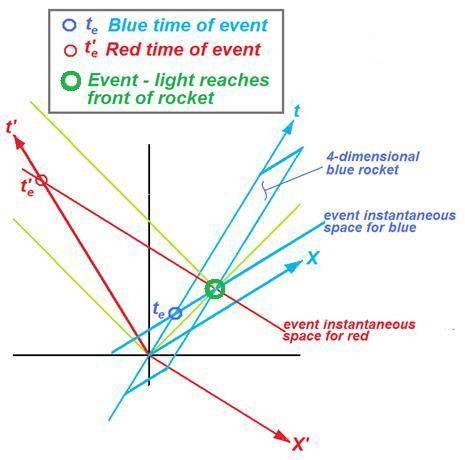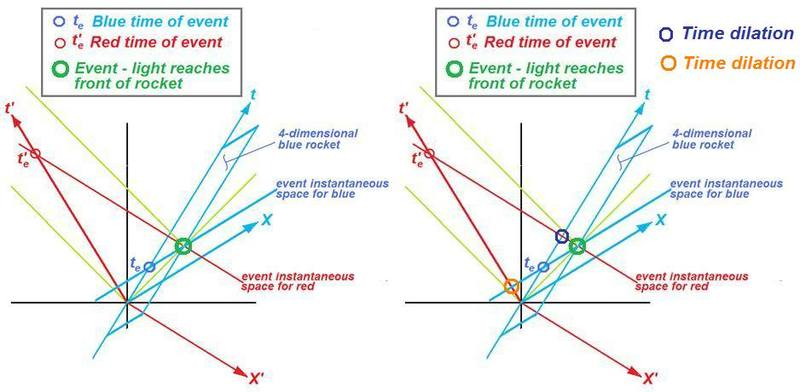# Inertial Frames in Relativity

If I am standing at the bottom a spaceship of a Length L moving at a certain speed v (let's say half light speed so that relativistic effects aren't negligible), how would I go about calculating how long it would take the light beam to reach the top of the spaceship?

In Newtonian mechanics, it would just be t =(vt + L)/c since I would have to account for the distance (vt) that the spaceship moves vertically while the light beam is moving to reach the top.

Would this be correct in Relativity also? I don't think I would be aware of any Lorentz contractions since I am unaware I am in a moving reference frame,right?

Janus
Staff Emeritus
Gold Member
If I am standing at the bottom a spaceship of a Length L moving at a certain speed v (let's say half light speed so that relativistic effects aren't negligible), how would I go about calculating how long it would take the light beam to reach the top of the spaceship?

In Newtonian mechanics, it would just be t =(vt + L)/c since I would have to account for the distance (vt) that the spaceship moves vertically while the light beam is moving to reach the top.

Would this be correct in Relativity also? I don't think I would be aware of any Lorentz contractions since I am unaware I am in a moving reference frame,right?

Light travels at c relative to any inertial frame as measured from that frame.

But for an observer standing on earth watching this, there would be time dilation and this event would appear to take longer,right?

Dale
Mentor
2020 Award
Time dilation doesn't apply to light beams. In the earth frame it would take longer simply because the light would travel a distance greater than L.

If I am standing at the bottom a spaceship of a Length L moving at a certain speed v (let's say half light speed so that relativistic effects aren't negligible), how would I go about calculating how long it would take the light beam to reach the top of the spaceship?

In Newtonian mechanics, it would just be t =(vt + L)/c since I would have to account for the distance (vt) that the spaceship moves vertically while the light beam is moving to reach the top.

Would this be correct in Relativity also? I don't think I would be aware of any Lorentz contractions since I am unaware I am in a moving reference frame,right?

Hi, Salamon

I won't do the calculations, but thought I'd give you a qualitative picture of what's going on. The sketch below is a symmetric spacetime diagram. That means I've chosen a reference frame of reference (black) for which the guy standing at the space ship (red) is moving to the left (with respect to the black coordinates) at relativistic speed, while the rocket (blue) is moving to the right at the very same relativistic speed. This allows you to have a spacetime diagram in which the red line lengths on the computer screen are calibrated to the same values as the blue lines (for blue t and red t' and for blue X and red X', respectively). The green lines at 45 degree angles are light photon world lines.

The blue rocket and red guy pass each other at zero time for both blue and red (they can synchronize their clocks to zero time as they pass). And the light beam turns on at zero time and arrives at the front of the rocket at the "event."Last edited:
Time dilation doesn't apply to light beams. In the earth frame it would take longer simply because the light would travel a distance greater than L.

In the sense that the light beam is moving in a parabolic curve (or some other type) in the reference frame of someone at rest relative to the ship?

Dale
Mentor
2020 Award
In the sense that the light beam is moving in a parabolic curve (or some other type) in the reference frame of someone at rest relative to the ship?
In the sense that the time dilation formula requires that the clock be at rest in one of the inertial frames, and a light beam is not at rest in any inertial frame. I.e. it simply doesn't apply.

In the sense that the light beam is moving in a parabolic curve (or some other type) in the reference frame of someone at rest relative to the ship?

That's why I used a symmetric spacetime diagram--so you wouldn't have to think about hypberbolic curves to see the time for blue relative to the time for red. In any case, the photons are going to be straight lines that bisect the angle between the time and 3-D spatial axis (X here) for any observer's inertial coordinates.

But DaleSpam is right. You are not trying to compute a Lorentz transformation for either te or te', you are trying to see the te and te' values for the event itself (light reaches the forward end of the rocket).

Of course you can look at the te and te' instantaneous spaces for the rocket and the red guy, respectively (see right side sketch below), and see the time dilations (qualitatively--I didn't do the arithmetic).Last edited:
jtbell
Mentor
In the sense that the light beam is moving in a parabolic curve (or some other type) in the reference frame of someone at rest relative to the ship?

In this situation the ship and the light beam are both moving in the same direction, so the light beam is a straight line in both frames.